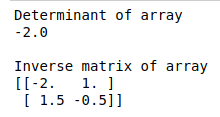• #5, First Floor, 4th Street Dr. Subbarayan Nagar Kodambakkam, Chennai-600 024 Landmark : Samiyar Madam
• pro@slogix.in
• +91- 81240 01111

#### Social List

##### How to find determinant and Inverse matrix of an array using scipy in python?
###### Description

To write a small piece of code for finding determinant and Inverse matrix using python scipy library.

#### Input :

2 dimensional array.

#### Output:

Determinant of 2D array.
Inverse matrix.

###### Process

Define a sample array.

Import linear algebra attributes from scipy.

Pass to the det() constructor.

Finds the determinant of an two dimensional array.

###### Sapmle Code

from scipy import linalg

import numpy as np

#define a 2-dimensional array

sample_array = np.array([[1,2],[3,4]])

#pass values to det() function

print(“Determinant of array”)

d = linalg.det(sample_array)

print(d,”\n”)

#Inverse matrix of array

print(“Inverse matrix of array”)

I = linalg.inv(sample_array)

print(I)

###### Screenshots